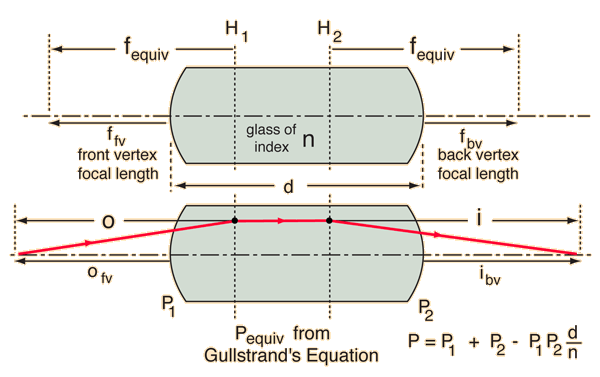# Image from Principal Planes

The thin lens equation can be used with thick lenses if the principal planes are found. The equivalent focal length of the thick lens can be found from Gullstrand's equation, and the front and back vertex focal lengths obtained. Once that is done, the principal planes can be located by subtracting the vertex focal lengths from the equivalent focal length. The equivalent focal length and the distance from first principal distance H1 to the object can be used to calculate the image distance from the second principal plane H2. From that distance, the image distance from the back vertex can be deduced.Note that the calculation below does not take into account the change in lens thickness with the angle of the incoming ray. It is typical to do the calculation only for the paraxial rays where the departure from full thickness is negligible. The segments in boxes below are the limiting cases where the lens thickness is taken as zero (thin lens approximation).

For glass with index of refraction nlens =

the front surface power for lens radius R1 = m is P1 = m-1

and the back surface power for lens radius R2 = m is P2 = m-1

(Note that for a double convex lens, the front surface radius R1 is positive and the back surface radius R2 is negative according to the Cartesian sign convention.)

 A thin lens would have approximate power Pthin lens = P1 + P2 = m-1. The thin lens focal length would be f = m = cm.

A thick lens of thickness d = cm = m would have effective power given by Gullstrand's equation:

Pthick lens = m-1

The equivalent focal length for the thick lens would be fequiv = m = cm.

Sometimes for thick lenses it is more useful to state the back vertex power and focal length. For this lens:

Pfront vertex = m-1.

Front vertex focal length = ffront vertex = m = cm.

Pback vertex = m-1.

Back vertex focal length = fback vertex = m = cm.

 For the thin lens, we can calculate the image distance from the simpler form of the lens equation. For object distance o = m, the image distance is i = m.

Remember that an object in front of the lens gives a negative object distance. Also remember that an object inside the focal length does not form a real image. A negative image distance impies a virtual image.

For the thick lens, we will specify the distance of the object from the front surface of the lens:

ofv = m.

To use the lens equation for the thick lens, we must find the object distance to the first principal plane H1, so we must calculate the distance from the front vertex to that plane. That distance is the difference between the equivalent focal length and the front vertex focal length, fequiv - ffv.

The distance from the front vertex to H1 = m so othick lens = m.

The lens equation, using Pthick lens, then gives the image distance

ithick lens = m.

This locates the image for us, but if we want the image distance from the back vertex, we have to calculate the distance from H2 to that vertex. That distance is the difference between the equivalent focal length and the back vertex focal length, fequiv - fbv.

H2 to back vertex = m.

The image distance from the back vertex is then

ibv = m.

Note that the use of the lens equation in this setting leaves out the separation between the principal planes.

H2 - H1 = m.

The total distance from the object to the image for the thick lens is

othick + (H2 - H1) + ithick = m.

 For the thin lens, this distance would be just o+i = m. In many practical cases this distance is very close to just the thick lens total distance minus the thickness of the lens.
 Compare to same calculation by: Vergence tracing
 Principal planes, two thin lenses Vergence Example Matrix definitions
Index

Lens concepts

Thick lens concepts

Reference
Meyer-Arendt

 HyperPhysics***** Light and Vision R Nave
Go Back